Published

# Team Angeldrew Katraline

We have coded an RC car to autonomously follow a track and stop at a "stop sign".

BeginnerShowcase (no instructions)67## Things used in this project

### Hardware components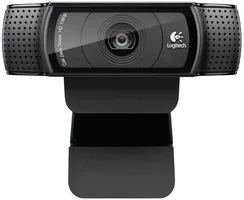Webcam, Logitech® HD Pro
×1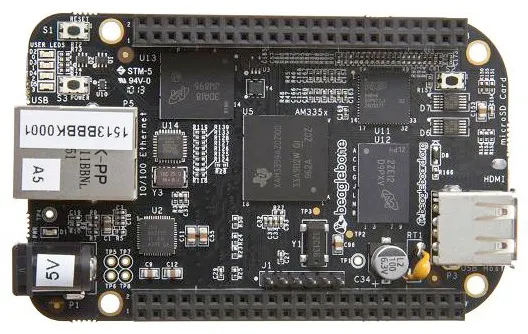BeagleBoard.org BeagleBone Black
×1USB Expansion HUB, USB 2.0 Powered
×1Test Accessory, USB Wi-Fi Dongle
×1

### Software apps and online servicesOpenCV

## Schematics

### Our Car Setup (Front View)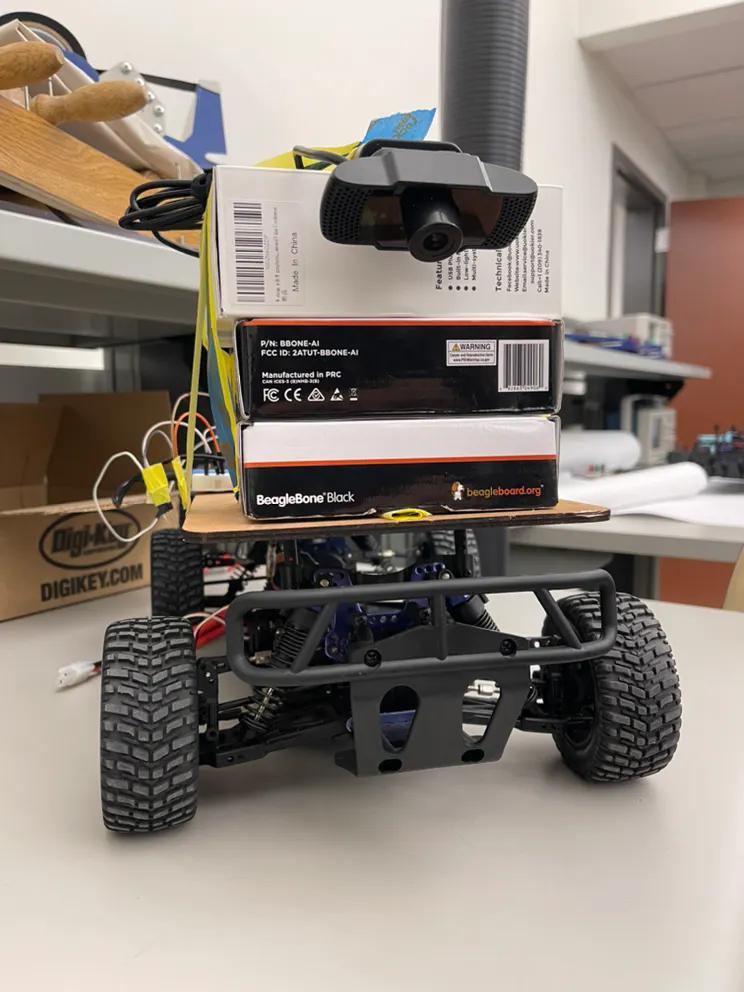### Our Car Setup (Top View)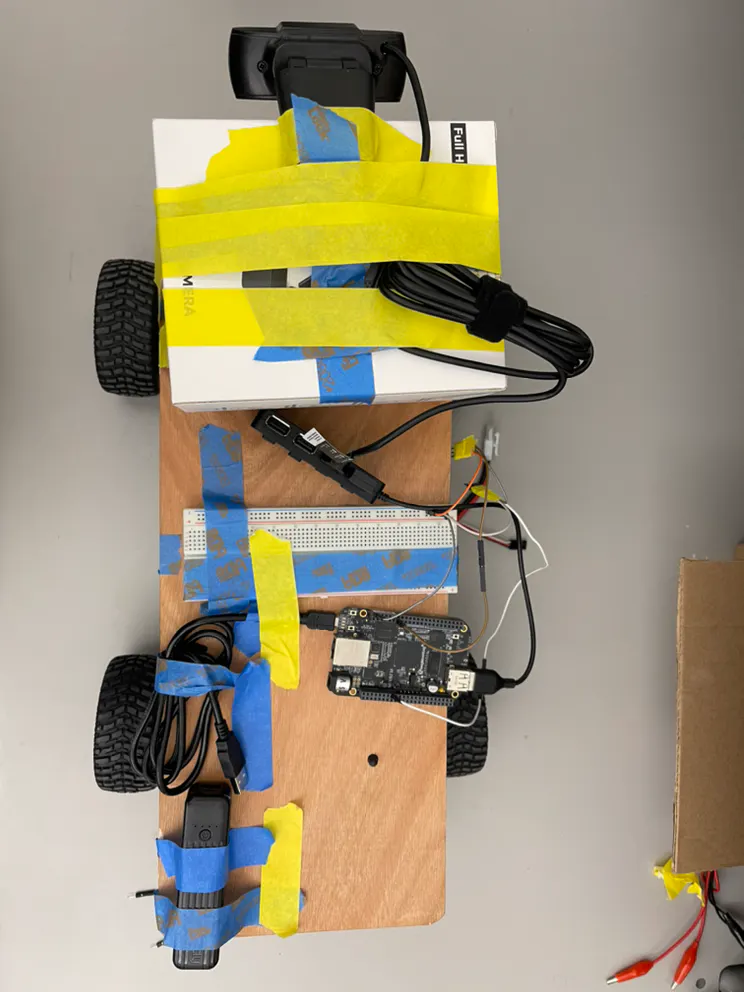### Graph of Steering, Throttle and Error vs Frame Number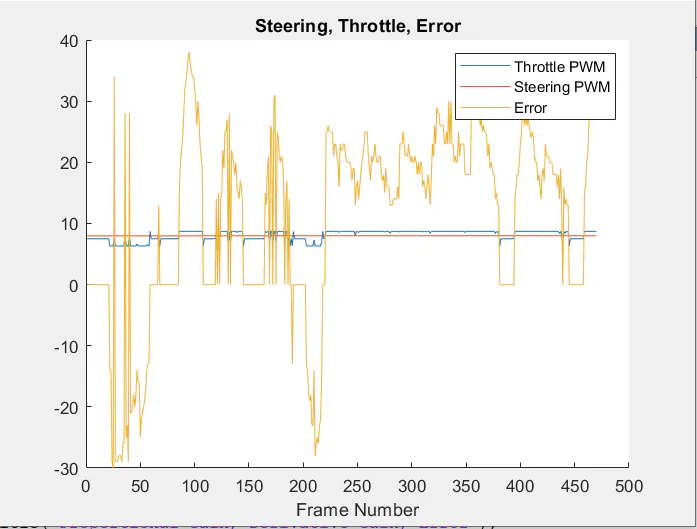### Graph of Proportional Gain, Derivative Gain and Error vs Frame Number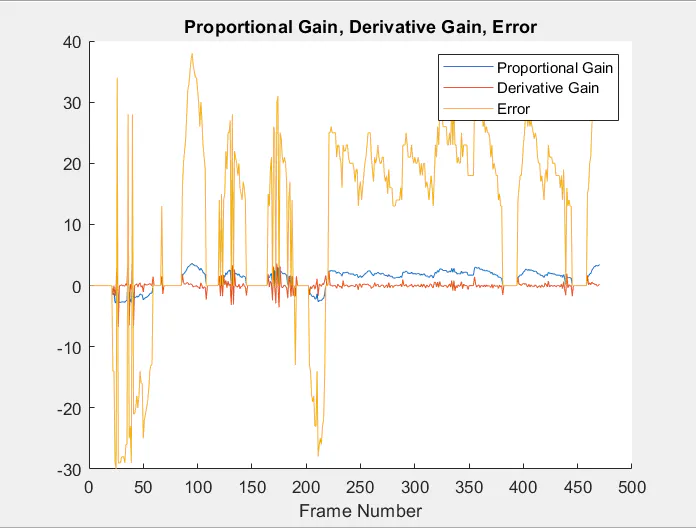## Code

### Code

Python
```# Code based on user raja_961's instructable found here:
# https://www.instructables.com/Autonomous-Lane-Keeping-Car-Using-Raspberry-Pi-and/

import cv2
import numpy as np
import math
import sys
import time
import Adafruit_BBIO.PWM as PWM

# GPIO.setwarnings(False)

#throttle = physical pin 66
throttlePin = "P8_13" # Physical pin 22
# in3 = 23 # physical Pin 16
# in4 = 24 # physical Pin 18

#Steering
steeringPin = "P9_16" # Physical Pin 15
# in1 = 17 # Physical Pin 11
# in2 = 27 # Physical Pin 13

# Throttle
PWM.start(throttlePin,7.5, 50)
#PWM.set_duty_cycle(throttlePin,7.5)
# PWM.stop(throttlePin)

# Steering
PWM.start(steeringPin,7.5, 50)
#PWM.set_duty_cycle(steeringPin, 7.5)
# PWM.stop(steeringPin)

def detect_stop(hsv):
#takes image in hsv as input

#mask for the lower hue values
red_lower = np.array([0,50,50], dtype = "uint8")
red_higher = np.array([10,255,255], dtype = "uint8")
#find pixels that are within the mask range
mask0 = cv2.inRange(hsv, red_lower, red_higher)
#mask for the higher hue values
red_lower = np.array([170,50,50], dtype = "uint8")
red_higher = np.array([180,255,255], dtype = "uint8")
#find pixels within upper mask range
mask1 = cv2.inRange(hsv, red_lower, red_higher)

#get all pixels that are red
#sum up all values (0 if not red, 255 = logical 1 if red)

#if more than 3/8's of total screen (3/4s of bottom half) is red -> there is a "stop sign"
#return true if stop sign, false otherwise
return True if totalRed >= 80*60/9*1*255 else False

def detect_edges(hsv):
#changed to have hsv as input so that it doesnt need to be computed for edges and stops
# filter for blue lane lines
#cv2.imshow("HSV",hsv)
lower_blue = np.array([90, 120, 0], dtype = "uint8")
upper_blue = np.array([150, 255, 255], dtype="uint8")

# detect edges
edges = cv2.Canny(mask, 50, 100)
#cv2.imshow("edges",edges)

return edges

def region_of_interest(frame):
#changed to find ROI from original frame
height, width, depth = frame.shape
cropped_frame = np.zeros_like(frame)
# only focus lower half of the screen
polygon = np.array([[
(0, height//2),
(width, height // 2),
(width, height - 1),
(0, height - 1)
]], np.int32)

# on image "mask", fill a square with corner points "polygon" with 1's
#blackens out top half of the screen
cropped_frame = cv2.bitwise_and(frame, mask)
# cv2.imshow("roi",cropped_frame)

return cropped_frame

def detect_line_segments(cropped_edges):
rho = 1
theta = np.pi / 180
min_threshold = 10

line_segments = cv2.HoughLinesP(cropped_edges, rho, theta, min_threshold,
np.array([]), minLineLength=5, maxLineGap=150)

return line_segments

def average_slope_intercept(frame, line_segments):
lane_lines = []

if line_segments is None:
#print("no line segments detected")
return lane_lines

height, width,_ = frame.shape
left_fit = []
right_fit = []

boundary = 1/3
left_region_boundary = width * (1 - boundary)
right_region_boundary = width * boundary

for line_segment in line_segments:
for x1, y1, x2, y2 in line_segment:
if x1 == x2:
#print("skipping vertical lines (slope = infinity")
continue

fit = np.polyfit((x1, x2), (y1, y2), 1)
slope = (y2 - y1) / (x2 - x1)
intercept = y1 - (slope * x1)

if slope < 0:
if x1 < left_region_boundary and x2 < left_region_boundary:
left_fit.append((slope, intercept))
else:
if x1 > right_region_boundary and x2 > right_region_boundary:
right_fit.append((slope, intercept))

left_fit_average = np.average(left_fit, axis=0)
if len(left_fit) > 0:
lane_lines.append(make_points(frame, left_fit_average))

right_fit_average = np.average(right_fit, axis=0)
if len(right_fit) > 0:
lane_lines.append(make_points(frame, right_fit_average))

return lane_lines

def make_points(frame, line):
height, width, _ = frame.shape

slope, intercept = line

y1 = height  # bottom of the frame
y2 = int(y1 / 2)  # make points from middle of the frame down

if slope == 0:
slope = 0.1

x1 = int((y1 - intercept) / slope)
x2 = int((y2 - intercept) / slope)

return [[x1, y1, x2, y2]]

def display_lines(frame, lines, line_color=(0, 255, 0), line_width=6):
line_image = np.zeros_like(frame)

if lines is not None:
for line in lines:
for x1, y1, x2, y2 in line:
cv2.line(line_image, (x1, y1), (x2, y2), line_color, line_width)

line_image = cv2.addWeighted(frame, 0.8, line_image, 1, 1)

return line_image

def display_heading_line(frame, steering_angle, line_color=(0, 0, 255), line_width=5 ):
height, width, _ = frame.shape

steering_angle_radian = steering_angle / 180.0 * math.pi

x1 = int(width / 2)
y1 = height
x2 = int(x1 - height / 2 / math.tan(steering_angle_radian))
y2 = int(height / 2)

cv2.line(heading_image, (x1, y1), (x2, y2), line_color, line_width)

def get_steering_angle(frame, lane_lines):

height,width,_ = frame.shape

if len(lane_lines) == 2:
_, _, left_x2, _ = lane_lines
_, _, right_x2, _ = lane_lines
mid = int(width / 2)
x_offset = (left_x2 + right_x2) / 2 - mid
y_offset = int(height / 2)

elif len(lane_lines) == 1:
x1, _, x2, _ = lane_lines
x_offset = x2 - x1
y_offset = int(height / 2)

elif len(lane_lines) == 0:
x_offset = 0
y_offset = int(height / 2)

angle_to_mid_radian = math.atan(x_offset / y_offset)
angle_to_mid_deg = int(angle_to_mid_radian * 180.0 / math.pi)
steering_angle = angle_to_mid_deg + 90

return steering_angle

video = cv2.VideoCapture(0)
video.set(cv2.CAP_PROP_FRAME_WIDTH,320)
video.set(cv2.CAP_PROP_FRAME_HEIGHT,240)

time.sleep(1)

##fourcc = cv2.VideoWriter_fourcc(*'XVID')
##out = cv2.VideoWriter('Original15.avi',fourcc,10,(320,240))
##out2 = cv2.VideoWriter('Direction15.avi',fourcc,10,(320,240))

speed = 7.96
lastTime = 0
lastError = 0

kp = 0.095
kd = kp * 0.07 #0.05
foundStop = False
red = 0
PWM.set_duty_cycle(throttlePin, speed)
PWM.set_duty_cycle(steeringPin, 7.5)

i = 0
while i < 300:
frame = cv2.resize(frame, (80, 60), interpolation=cv2.INTER_AREA)
# frame = cv2.flip(frame,-1)
if red > 1:
break
#cv2.imshow("original",frame)
#computed first so it doesnt have to be found each time for edges and stop sign detection
roi = region_of_interest(frame)
#moved outside detect edges to only convert roi frame once
hsv = cv2.cvtColor(roi, cv2.COLOR_BGR2HSV)

#check for stops
if detect_stop(hsv):
#makes sure it's detecting stop for the first time
#sleep for 3 seconds and set the speed to 0
PWM.set_duty_cycle(throttlePin, 7.5)
time.sleep(3)
#notes that we detected it for the first time, will not run again until another stop sign
foundStop = True
speed += .025
#print("STOOOOOOOOOOOOOOOOP")
red += 1
continue
else:
PWM.set_duty_cycle(throttlePin,speed)

#if no stop sign but foundStop is true -> we just passed a stop sign
elif foundStop:
#set foundStop to false for the next time
foundStop = False

PWM.set_duty_cycle(throttlePin,speed)
# print(i)
edges = detect_edges(hsv)
line_segments = detect_line_segments(edges)
lane_lines = average_slope_intercept(frame,line_segments)
lane_lines_image = display_lines(frame,lane_lines)
steering_angle = get_steering_angle(frame, lane_lines)

now = time.time()
dt = now - lastTime

deviation = steering_angle - 90
error = -1*deviation

if deviation < 13 and deviation > -13:
deviation = 0
error = 0
#    PWM.set_duty_cycle(steeringPin,7.5)

# elif deviation > 5:
#    PWM.set_duty_cycle(steeringPin,7.2)

# elif deviation < -5:
#     PWM.set_duty_cycle(steeringPin,7.9)

derivative = kd * (error - lastError) / dt
proportional = kp * (error)
PD = 7.5 + derivative + proportional
if PD > 8.7:
PD = 8.7
if PD < 6.3:
PD = 6.3

print(PD, speed, error, derivative, proportional)
if abs(error - lastError) <= 10 and i < 100: # 5
lastError = error
lastTime = time.time()
continue

PWM.set_duty_cycle(steeringPin,PD)
if abs(PD- 7.5) > .80:
PWM.set_duty_cycle(throttlePin,speed-.005)
else:
PWM.set_duty_cycle(throttlePin,speed)
lastError = error
lastTime = time.time()

#    out.write(frame)
i += 1
key = cv2.waitKey(1)
if key == 27:
break

PWM.set_duty_cycle(throttlePin,7.5)
PWM.set_duty_cycle(steeringPin,7.5)
#print("Reseted")

PWM.stop(throttlePin)
PWM.stop(steeringPin)
video.release()
##out.release()
##out2.release()
cv2.destroyAllWindows()
PWM.cleanup()
```

## Credits

2 projects • 1 follower

### Katrina Ji

2 projects • 0 followers

### Angela Liu

2 projects • 1 follower

### Andrew Yan

2 projects • 1 follower